# Liquids and Solids Chapter 13 Solids Liquids and

• Slides: 67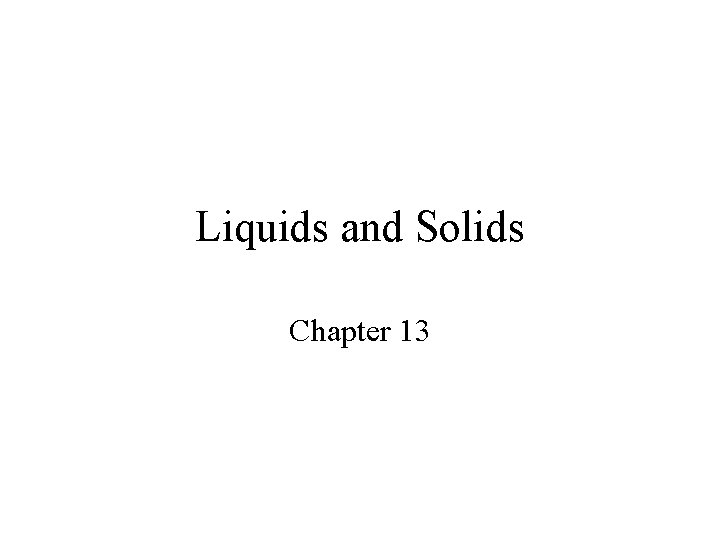Liquids and Solids Chapter 13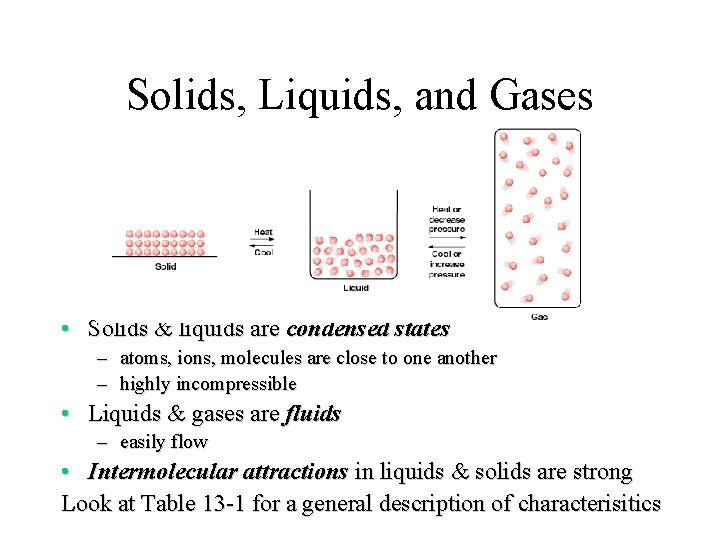Solids, Liquids, and Gases • Solids & liquids are condensed states – atoms, ions, molecules are close to one another – highly incompressible • Liquids & gases are fluids – easily flow • Intermolecular attractions in liquids & solids are strong Look at Table 13 -1 for a general description of characterisitics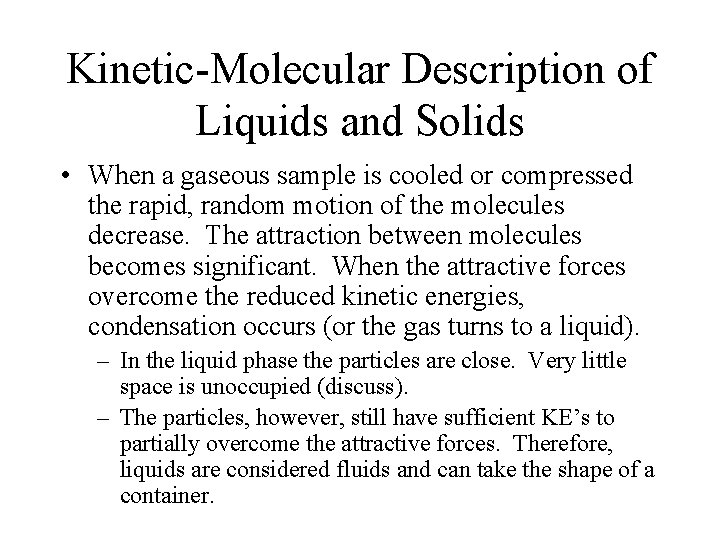Kinetic-Molecular Description of Liquids and Solids • When a gaseous sample is cooled or compressed the rapid, random motion of the molecules decrease. The attraction between molecules becomes significant. When the attractive forces overcome the reduced kinetic energies, condensation occurs (or the gas turns to a liquid). – In the liquid phase the particles are close. Very little space is unoccupied (discuss). – The particles, however, still have sufficient KE’s to partially overcome the attractive forces. Therefore, liquids are considered fluids and can take the shape of a container.Kinetic-Molecular Description of Liquids & Solids • Liquids that diffuse into one another are miscible (i. e. one liquid is soluble in the other) – Water and methanol/gasoline and motor oil • Immiscible liquid do not diffuse into one another (i. e. one liquid is not soluble in the other) – Water and hexane/water and gasoline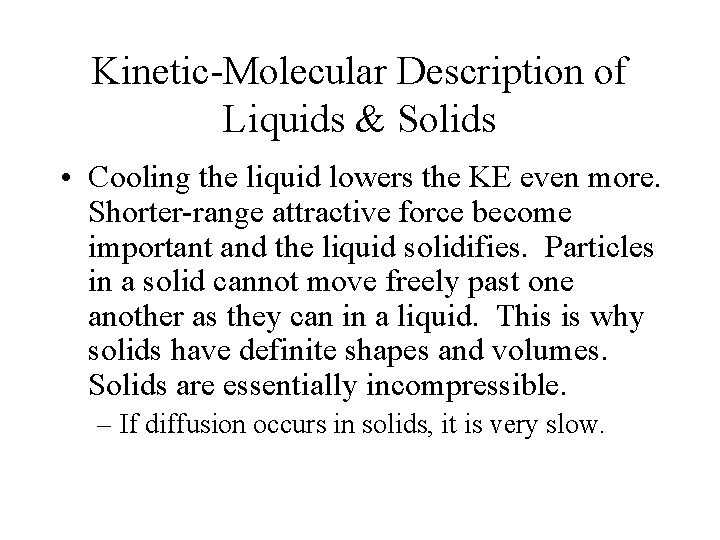Kinetic-Molecular Description of Liquids & Solids • Cooling the liquid lowers the KE even more. Shorter-range attractive force become important and the liquid solidifies. Particles in a solid cannot move freely past one another as they can in a liquid. This is why solids have definite shapes and volumes. Solids are essentially incompressible. – If diffusion occurs in solids, it is very slow.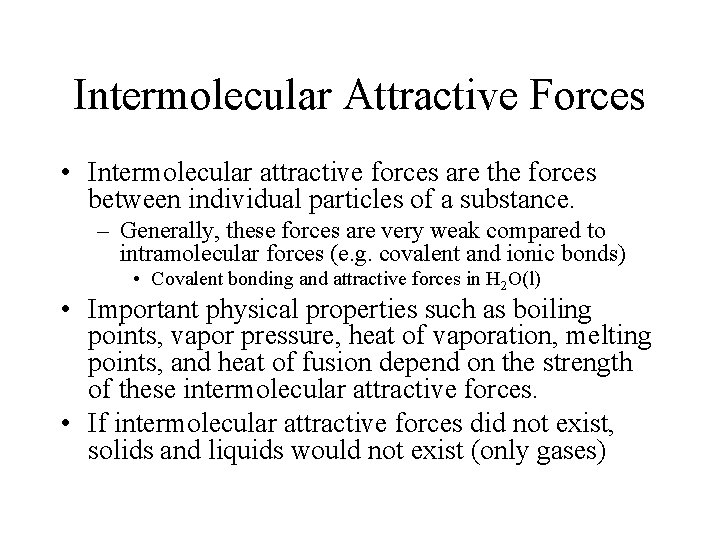Intermolecular Attractive Forces • Intermolecular attractive forces are the forces between individual particles of a substance. – Generally, these forces are very weak compared to intramolecular forces (e. g. covalent and ionic bonds) • Covalent bonding and attractive forces in H 2 O(l) • Important physical properties such as boiling points, vapor pressure, heat of vaporation, melting points, and heat of fusion depend on the strength of these intermolecular attractive forces. • If intermolecular attractive forces did not exist, solids and liquids would not exist (only gases)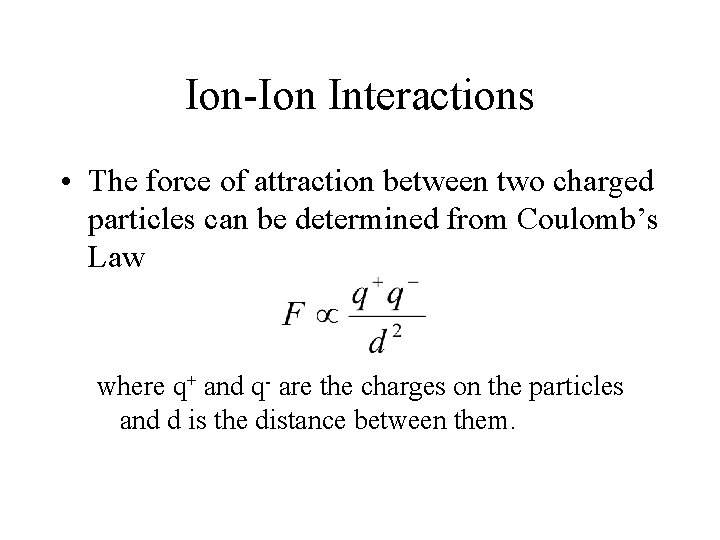Ion-Ion Interactions • The force of attraction between two charged particles can be determined from Coulomb’s Law where q+ and q- are the charges on the particles and d is the distance between them.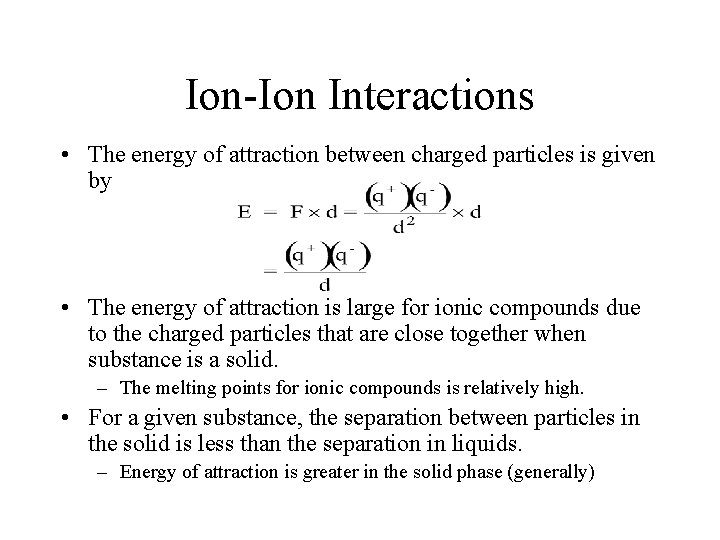Ion-Ion Interactions • The energy of attraction between charged particles is given by • The energy of attraction is large for ionic compounds due to the charged particles that are close together when substance is a solid. – The melting points for ionic compounds is relatively high. • For a given substance, the separation between particles in the solid is less than the separation in liquids. – Energy of attraction is greater in the solid phase (generally)Ion-Ion Interactions • Ionic compounds that possess multiplycharged ions (e. g. Al 3+ and O 2 -) usually have higher melting points than ionic compounds that posses singly-charged particles. Why (two reasons)? • Arrange the following ionic compounds in the expected order of increasing melting and boiling points. Na. F, Ca. O, Ca. F 2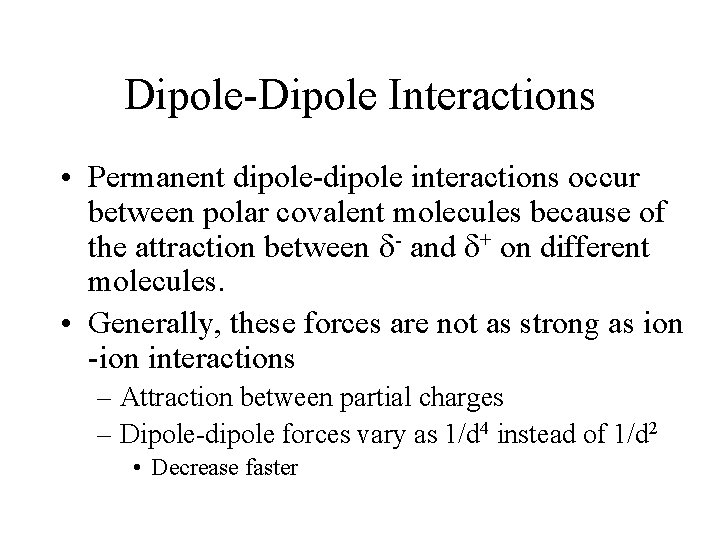Dipole-Dipole Interactions • Permanent dipole-dipole interactions occur between polar covalent molecules because of the attraction between - and + on different molecules. • Generally, these forces are not as strong as ion -ion interactions – Attraction between partial charges – Dipole-dipole forces vary as 1/d 4 instead of 1/d 2 • Decrease fasterDipole-Dipole Interactions The partial positive charge on the hydrogen atoms is attracted to the partial negative charge on the nitrogen atoms. Note: Dipole-dipole interactions are dependent on temperature. Why?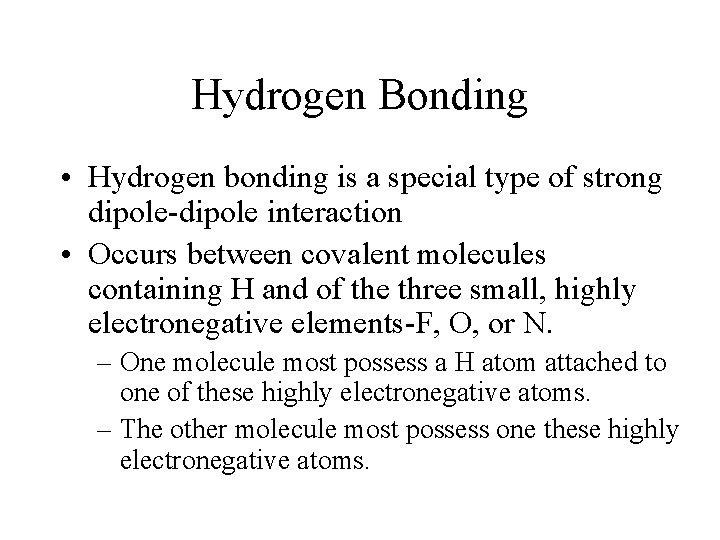Hydrogen Bonding • Hydrogen bonding is a special type of strong dipole-dipole interaction • Occurs between covalent molecules containing H and of the three small, highly electronegative elements-F, O, or N. – One molecule most possess a H atom attached to one of these highly electronegative atoms. – The other molecule most possess one these highly electronegative atoms.Hydrogen Bonding The partial negative charge of one molecule is attracted to the partial positive charge of another molecule. The small sizes of F, O, and N and their high electronegativities concentrate the electrons around these atoms.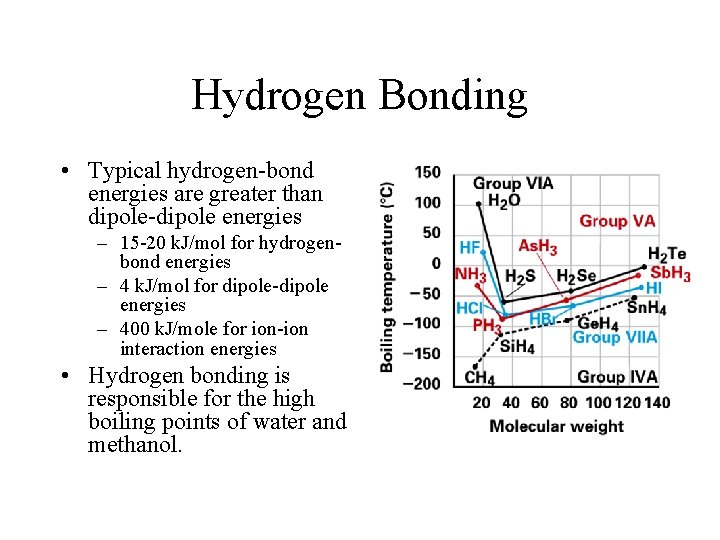Hydrogen Bonding • Typical hydrogen-bond energies are greater than dipole-dipole energies – 15 -20 k. J/mol for hydrogenbond energies – 4 k. J/mol for dipole-dipole energies – 400 k. J/mole for ion-ion interaction energies • Hydrogen bonding is responsible for the high boiling points of water and methanol.Dispersion Forces • These are attractive forces that are present in all types of molecules. – Dispersion forces are weak in small molecules. – They are important at extremely small distances which vary as 1/d 7. – They are the only attractive force present in symmetrical nonpolar substances such as Cl 2 and monatomic species.Dispersion Forces • Dispersion forces result from the attraction of a positively charged nucleus to the electron cloud of another atom in nearby molecules. As a result, temporary dipoles are induced in the neighboring atoms or molecules. • The magnitude of the temporary dipole increases with increasing size of the electron cloud (or size of the molecule). The larger electron cloud is more diffuse and easily distorted. Adjacent molecules are polarized by the adjacent nuclei. Polarizibility increases with increasing sizes of molecules. Dispersion forces are, therefore, stronger for molecules that are larger or have more electrons.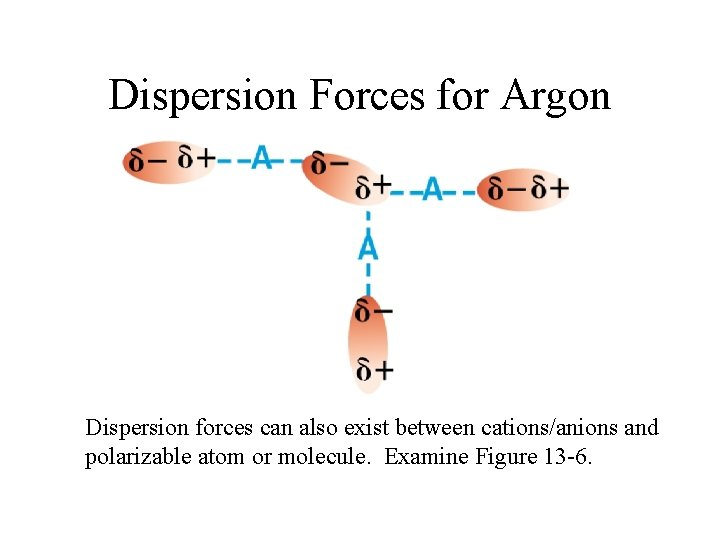Dispersion Forces for Argon Dispersion forces can also exist between cations/anions and polarizable atom or molecule. Examine Figure 13 -6.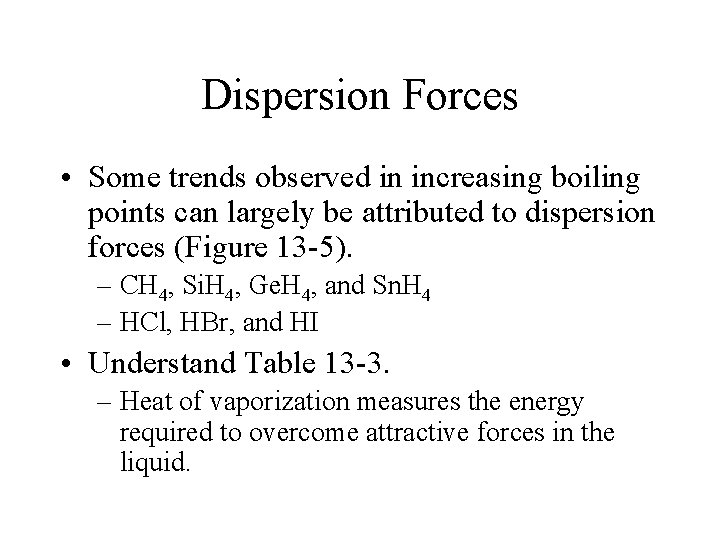Dispersion Forces • Some trends observed in increasing boiling points can largely be attributed to dispersion forces (Figure 13 -5). – CH 4, Si. H 4, Ge. H 4, and Sn. H 4 – HCl, HBr, and HI • Understand Table 13 -3. – Heat of vaporization measures the energy required to overcome attractive forces in the liquid.The Liquid State • Viscosity - the resistance to flow of a liquid. – Generally, the higher the attractive forces in a liquid, the greater the viscosity. • Water versus honey or Karo syrup. • Pentane versus dodecane – Viscosity decreases with increasing temperature. Why?The Liquid State • Surface Tension - measure of the inward forces that must be overcome to expand the surface area of a liquid. – Molecules at the surface are attracted unevenly. • Water bugs and floating razor blades (or needles) Demo: Razor blade or needleLiquid State • Capillary action - ability of a liquid to rise or fall in a tube. – Cohesive forces – forces holding a liquid together. – Adhesive force – forces between a liquid another surface – Capillary action • Capillary rise implies adhesive forces > cohesive forces • Capillary fall implies cohesive forces > adhesive forces Water is attracted to a glass capillary tube due to attractive forces between the partial negative oxygens on the surface of the glass and the partial positive charges on the hydrogen atoms.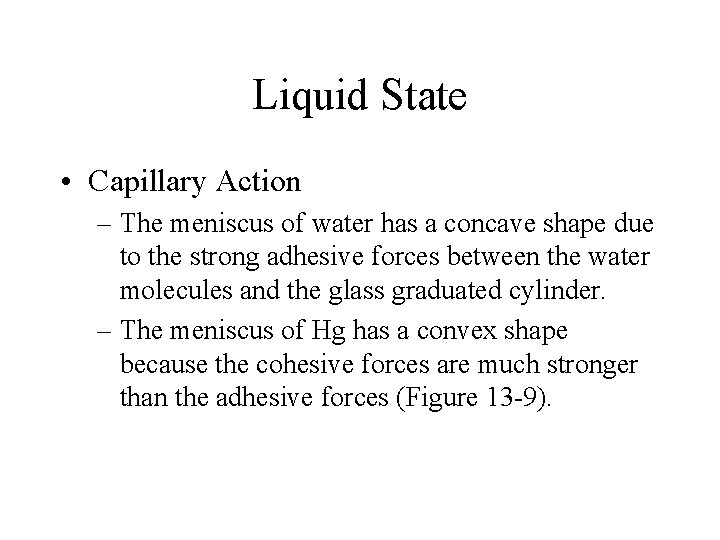Liquid State • Capillary Action – The meniscus of water has a concave shape due to the strong adhesive forces between the water molecules and the glass graduated cylinder. – The meniscus of Hg has a convex shape because the cohesive forces are much stronger than the adhesive forces (Figure 13 -9).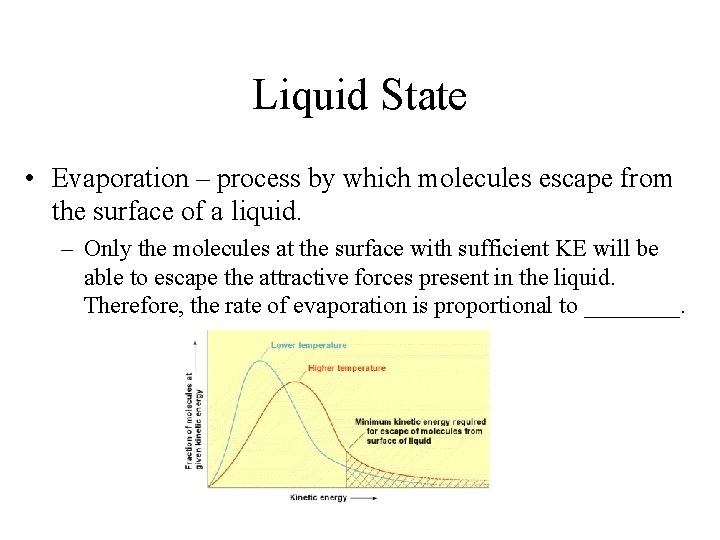Liquid State • Evaporation – process by which molecules escape from the surface of a liquid. – Only the molecules at the surface with sufficient KE will be able to escape the attractive forces present in the liquid. Therefore, the rate of evaporation is proportional to ____.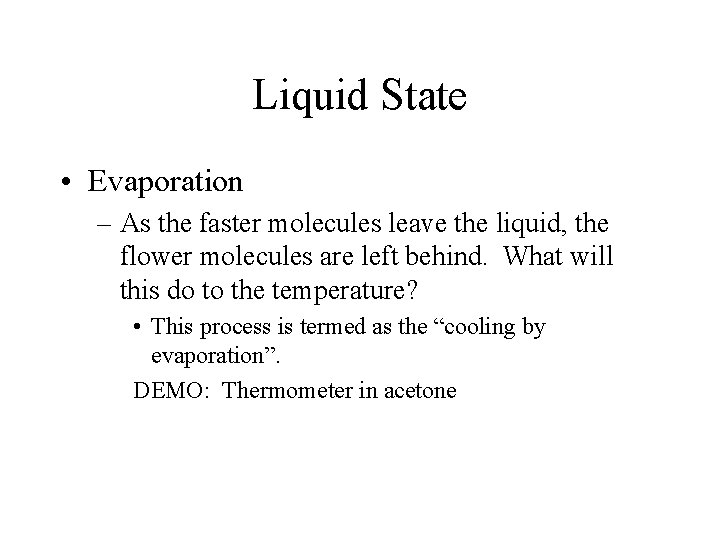Liquid State • Evaporation – As the faster molecules leave the liquid, the flower molecules are left behind. What will this do to the temperature? • This process is termed as the “cooling by evaporation”. DEMO: Thermometer in acetoneLiquid State • Evaporation and condensation – In an open container/beaker, all the water that is present will eventually escape into the gaseous phase (Figure 1310). – What if the beaker is sealed? What will happen? After molecules enter the gas phase they may be recaptured by the liquid by collisions. This process is called _______. At some point in time, the amount of gaseous molecule leaving the gaseous phase will equal the amount reentering the liquid phase. This is termed as dynamic equilibrium.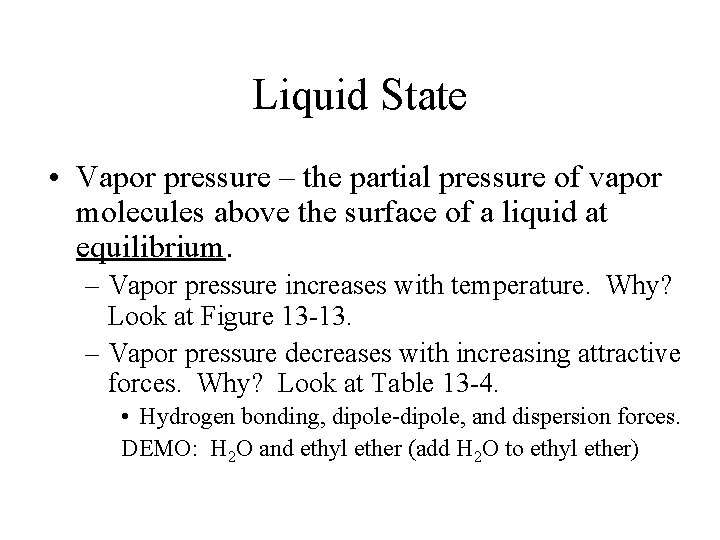Liquid State • Vapor pressure – the partial pressure of vapor molecules above the surface of a liquid at equilibrium. – Vapor pressure increases with temperature. Why? Look at Figure 13 -13. – Vapor pressure decreases with increasing attractive forces. Why? Look at Table 13 -4. • Hydrogen bonding, dipole-dipole, and dispersion forces. DEMO: H 2 O and ethyl ether (add H 2 O to ethyl ether)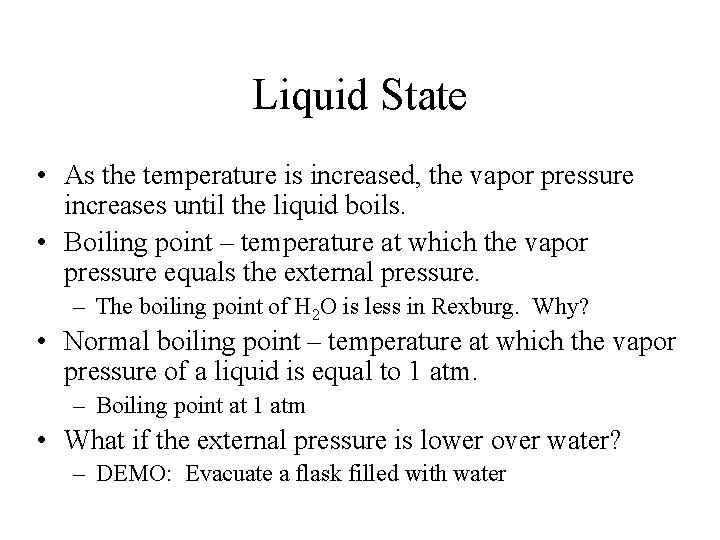Liquid State • As the temperature is increased, the vapor pressure increases until the liquid boils. • Boiling point – temperature at which the vapor pressure equals the external pressure. – The boiling point of H 2 O is less in Rexburg. Why? • Normal boiling point – temperature at which the vapor pressure of a liquid is equal to 1 atm. – Boiling point at 1 atm • What if the external pressure is lower over water? – DEMO: Evacuate a flask filled with water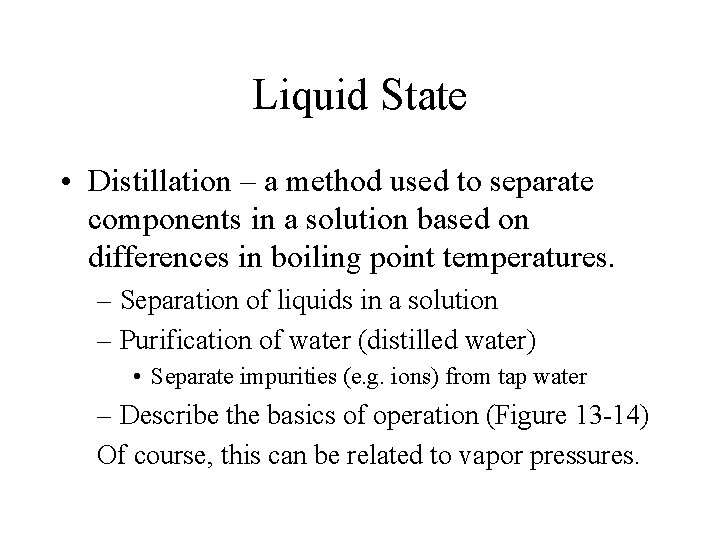Liquid State • Distillation – a method used to separate components in a solution based on differences in boiling point temperatures. – Separation of liquids in a solution – Purification of water (distilled water) • Separate impurities (e. g. ions) from tap water – Describe the basics of operation (Figure 13 -14) Of course, this can be related to vapor pressures.The Liquid State • Heat transfer involving liquids • Specific heat or molar heat capacity of a liquid is the amount of heat that must be added to 1 gram or 1 mole to raise the temperature by 1 C. How much heat is released by 200 g of H 2 O as it cools from 85. 0 o. C to 40. 0 o. C? The specific heat of water is 4. 184 J/go. C. Review in Chapter 1 if needed.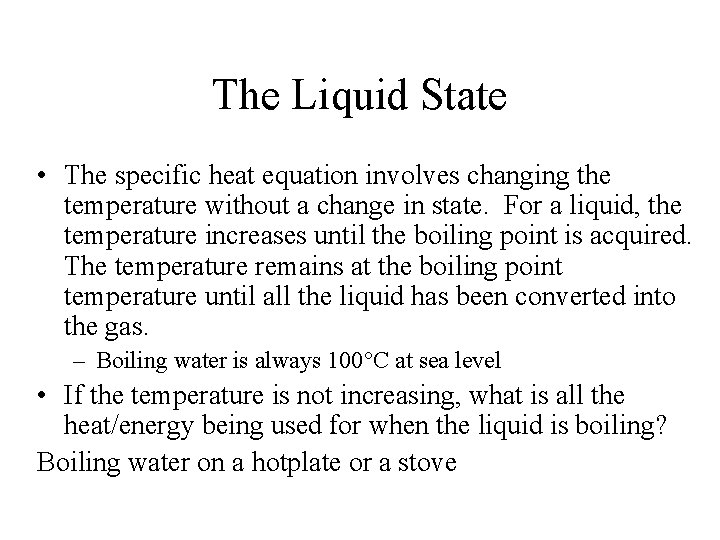The Liquid State • The specific heat equation involves changing the temperature without a change in state. For a liquid, the temperature increases until the boiling point is acquired. The temperature remains at the boiling point temperature until all the liquid has been converted into the gas. – Boiling water is always 100 C at sea level • If the temperature is not increasing, what is all the heat/energy being used for when the liquid is boiling? Boiling water on a hotplate or a stove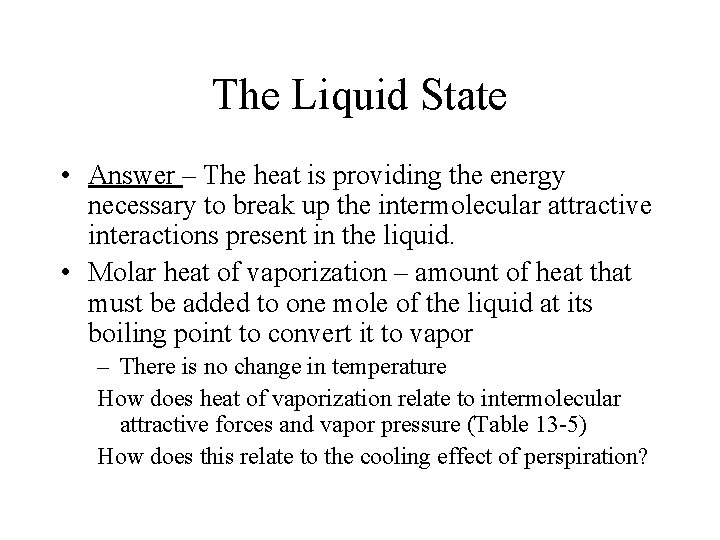The Liquid State • Answer – The heat is providing the energy necessary to break up the intermolecular attractive interactions present in the liquid. • Molar heat of vaporization – amount of heat that must be added to one mole of the liquid at its boiling point to convert it to vapor – There is no change in temperature How does heat of vaporization relate to intermolecular attractive forces and vapor pressure (Table 13 -5) How does this relate to the cooling effect of perspiration?The Liquid State • Condensation is the reverse of vaporization/evaporation. • Heat of condensation is the amount of heat that must be removed from a vapor to condense it. – Heat is released when the substance condenses.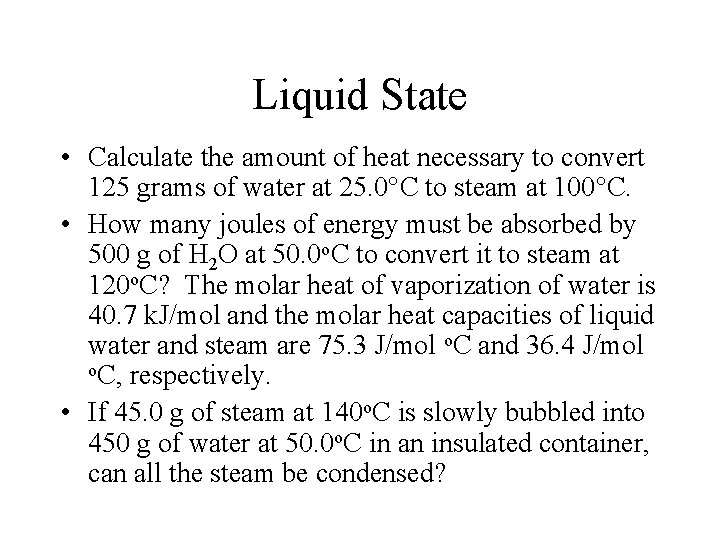Liquid State • Calculate the amount of heat necessary to convert 125 grams of water at 25. 0 C to steam at 100 C. • How many joules of energy must be absorbed by 500 g of H 2 O at 50. 0 o. C to convert it to steam at 120 o. C? The molar heat of vaporization of water is 40. 7 k. J/mol and the molar heat capacities of liquid water and steam are 75. 3 J/mol o. C and 36. 4 J/mol o. C, respectively. • If 45. 0 g of steam at 140 o. C is slowly bubbled into 450 g of water at 50. 0 o. C in an insulated container, can all the steam be condensed?Liquid State • Many trends in physical properties can be explained form the strength of intermolecular attractive forces in the liquid. – Table 13 -6 (understand these trends based on intermolecular attractive forces) • Arrange the following substances in order of increasing boiling points. – C 2 H 6, NH 3, Ar, Na. Cl, As. H 3 – Discuss trends on Figure 13 -5 (others as required) • Generally, if a group of molecules possess the same type of attractive forces, the boiling point increases with size/molecular weight of the compound.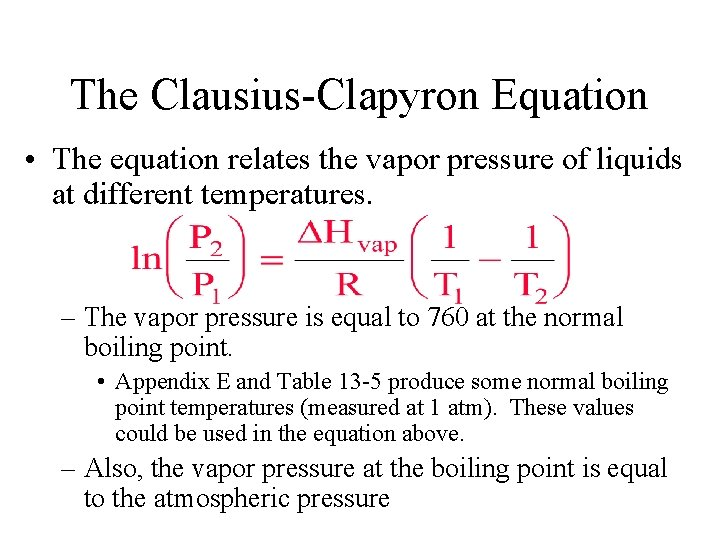The Clausius-Clapyron Equation • The equation relates the vapor pressure of liquids at different temperatures. – The vapor pressure is equal to 760 at the normal boiling point. • Appendix E and Table 13 -5 produce some normal boiling point temperatures (measured at 1 atm). These values could be used in the equation above. – Also, the vapor pressure at the boiling point is equal to the atmospheric pressure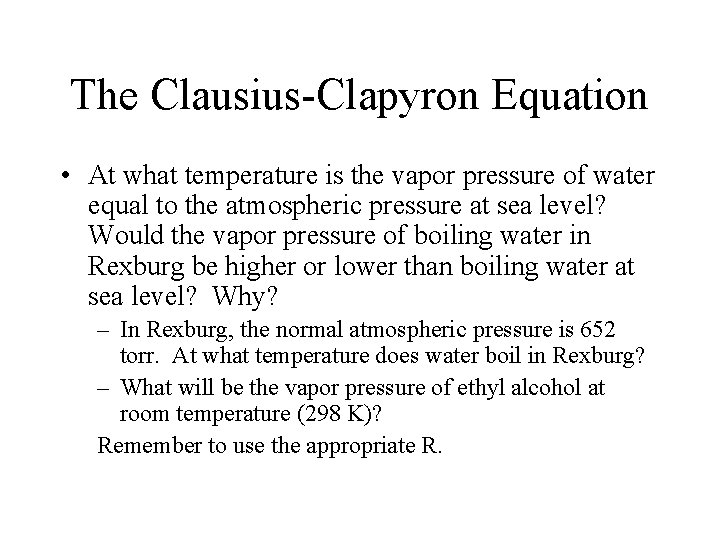The Clausius-Clapyron Equation • At what temperature is the vapor pressure of water equal to the atmospheric pressure at sea level? Would the vapor pressure of boiling water in Rexburg be higher or lower than boiling water at sea level? Why? – In Rexburg, the normal atmospheric pressure is 652 torr. At what temperature does water boil in Rexburg? – What will be the vapor pressure of ethyl alcohol at room temperature (298 K)? Remember to use the appropriate R.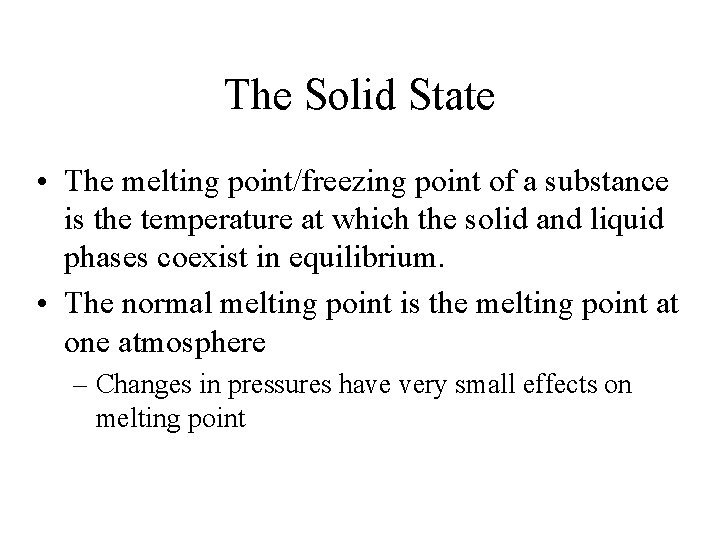The Solid State • The melting point/freezing point of a substance is the temperature at which the solid and liquid phases coexist in equilibrium. • The normal melting point is the melting point at one atmosphere – Changes in pressures have very small effects on melting point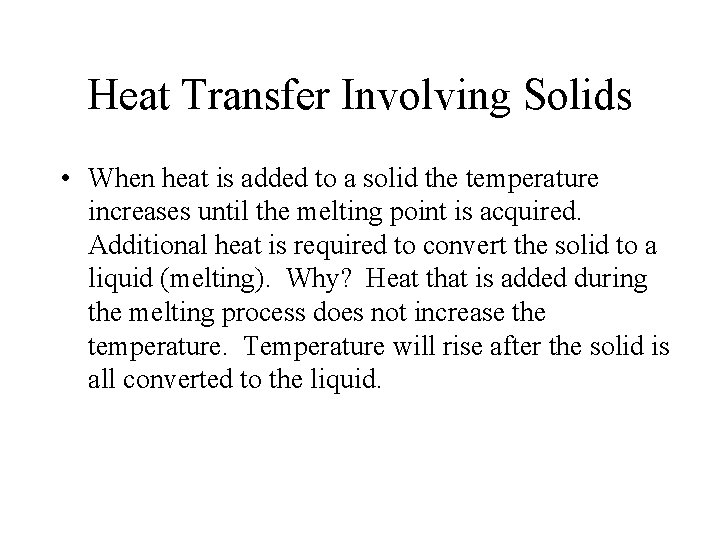Heat Transfer Involving Solids • When heat is added to a solid the temperature increases until the melting point is acquired. Additional heat is required to convert the solid to a liquid (melting). Why? Heat that is added during the melting process does not increase the temperature. Temperature will rise after the solid is all converted to the liquid.Heat Transfer Involving Solids • Molar heat of fusion ( Hfus; k. J/mol) is the amount of heat required to melt one mole of a solid at its melting point. This can be converted to heat of fusion which is (k. J/g) – Heat of fusion depends on _____(Table 13 -7) • Molar heat of solidification/crystallization is equal in magnitude (but opposite sign) to the molar heat of fusion. – 6. 02 k. J is absorbed when 1 mole is melted and 6. 02 k. J is released when 1 mole is frozen/solidifies.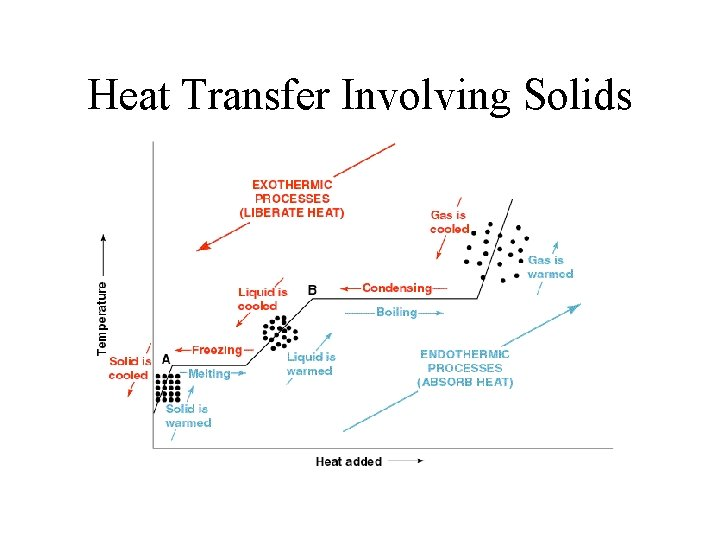Heat Transfer Involving Solids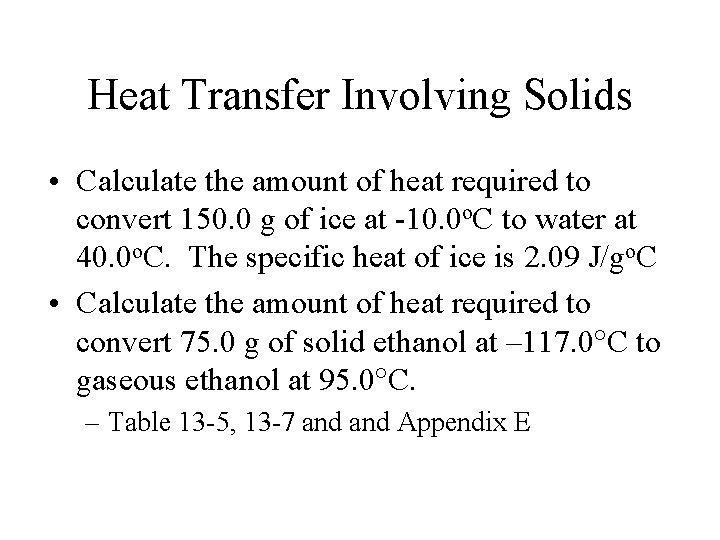Heat Transfer Involving Solids • Calculate the amount of heat required to convert 150. 0 g of ice at -10. 0 o. C to water at 40. 0 o. C. The specific heat of ice is 2. 09 J/go. C • Calculate the amount of heat required to convert 75. 0 g of solid ethanol at – 117. 0 C to gaseous ethanol at 95. 0 C. – Table 13 -5, 13 -7 and Appendix E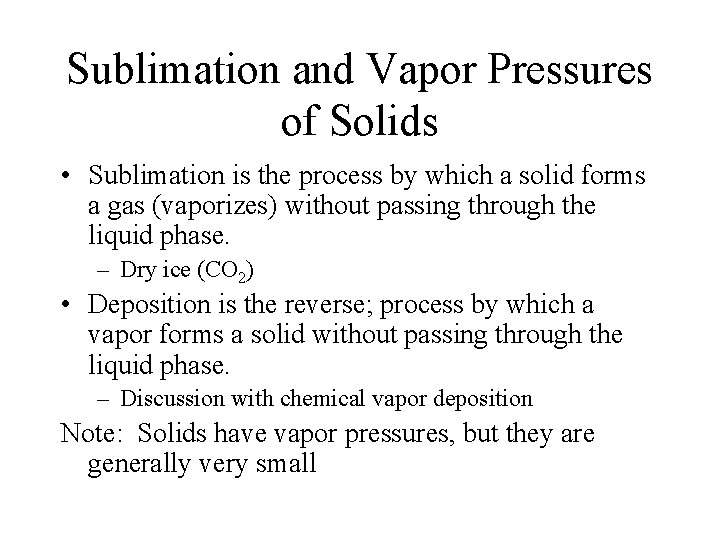Sublimation and Vapor Pressures of Solids • Sublimation is the process by which a solid forms a gas (vaporizes) without passing through the liquid phase. – Dry ice (CO 2) • Deposition is the reverse; process by which a vapor forms a solid without passing through the liquid phase. – Discussion with chemical vapor deposition Note: Solids have vapor pressures, but they are generally very smallPhase Diagrams (P versus T) • Phase diagrams illustrate a particular phase or state that is present under specific temperature-pressure conditions. – Temperature is on the y-axis and pressure is on the x-axis. • Using a phase diagram, changes in state can be determined when changing pressure and/or temperature.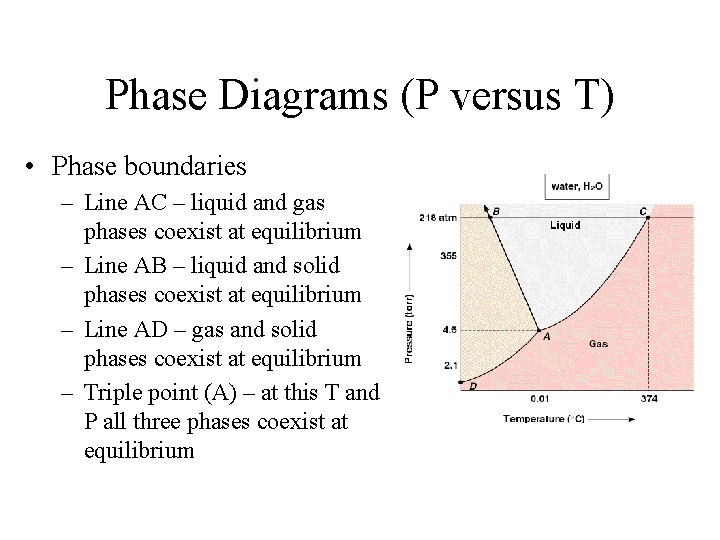Phase Diagrams (P versus T) • Phase boundaries – Line AC – liquid and gas phases coexist at equilibrium – Line AB – liquid and solid phases coexist at equilibrium – Line AD – gas and solid phases coexist at equilibrium – Triple point (A) – at this T and P all three phases coexist at equilibriumPhase Diagrams (P versus T) • Notice the negative AB line slope. This is unique to H 2 O. What happens upon compressing a solid (up from point J) • Is there a pressure where sublimation could occur? • Travel a few other lines.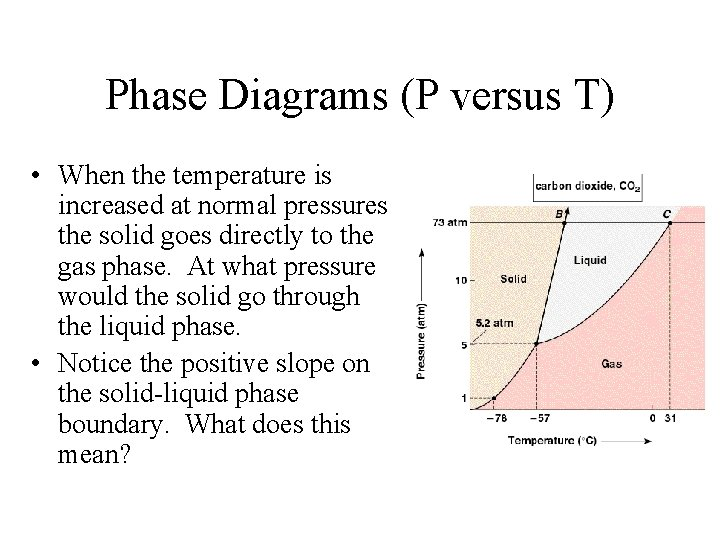Phase Diagrams (P versus T) • When the temperature is increased at normal pressures the solid goes directly to the gas phase. At what pressure would the solid go through the liquid phase. • Notice the positive slope on the solid-liquid phase boundary. What does this mean?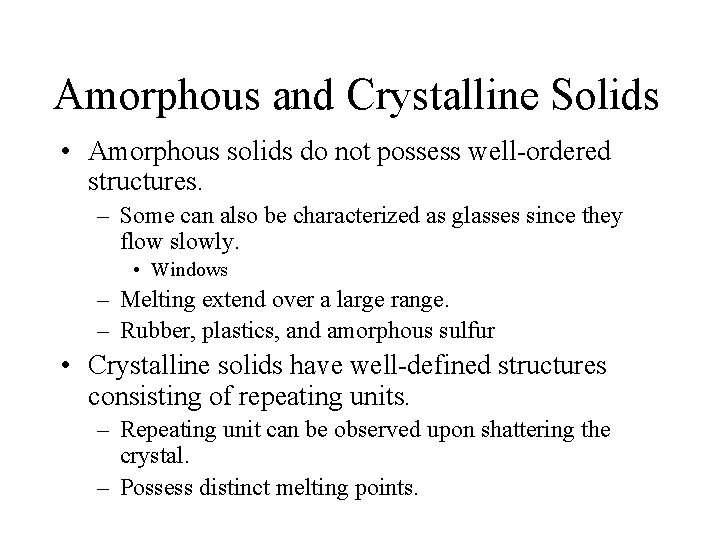Amorphous and Crystalline Solids • Amorphous solids do not possess well-ordered structures. – Some can also be characterized as glasses since they flow slowly. • Windows – Melting extend over a large range. – Rubber, plastics, and amorphous sulfur • Crystalline solids have well-defined structures consisting of repeating units. – Repeating unit can be observed upon shattering the crystal. – Possess distinct melting points.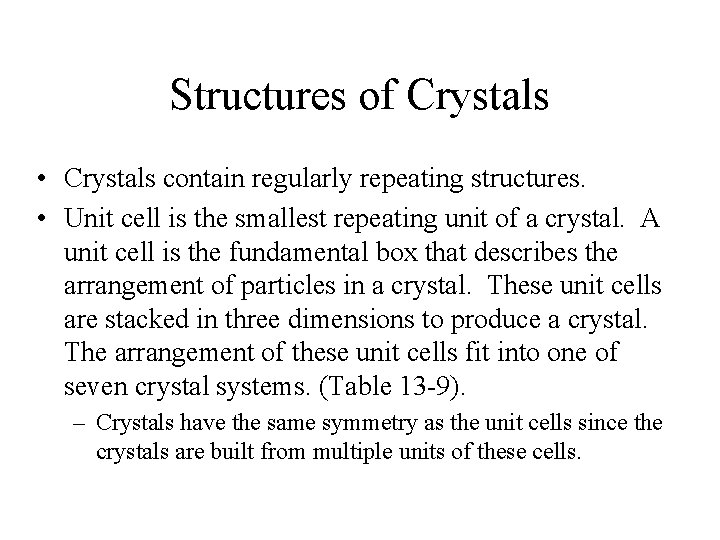Structures of Crystals • Crystals contain regularly repeating structures. • Unit cell is the smallest repeating unit of a crystal. A unit cell is the fundamental box that describes the arrangement of particles in a crystal. These unit cells are stacked in three dimensions to produce a crystal. The arrangement of these unit cells fit into one of seven crystal systems. (Table 13 -9). – Crystals have the same symmetry as the unit cells since the crystals are built from multiple units of these cells.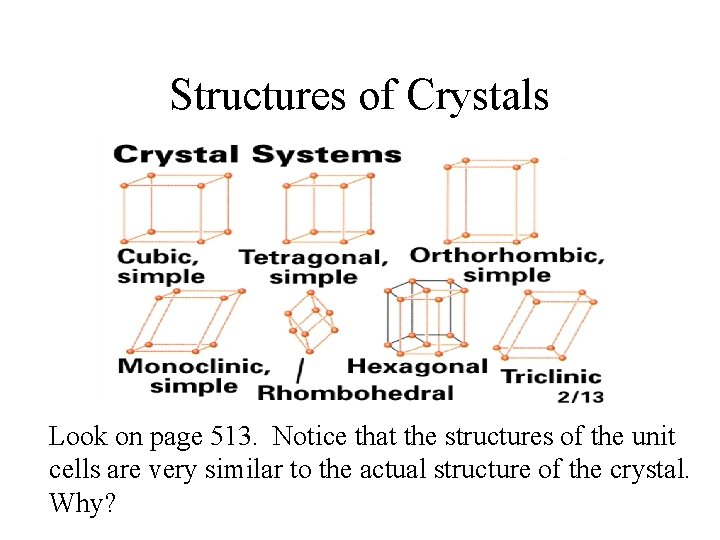Structures of Crystals Look on page 513. Notice that the structures of the unit cells are very similar to the actual structure of the crystal. Why?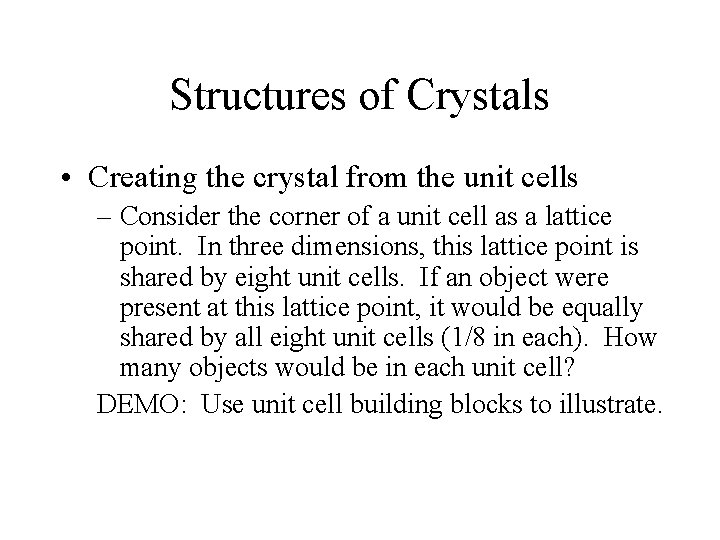Structures of Crystals • Creating the crystal from the unit cells – Consider the corner of a unit cell as a lattice point. In three dimensions, this lattice point is shared by eight unit cells. If an object were present at this lattice point, it would be equally shared by all eight unit cells (1/8 in each). How many objects would be in each unit cell? DEMO: Use unit cell building blocks to illustrate.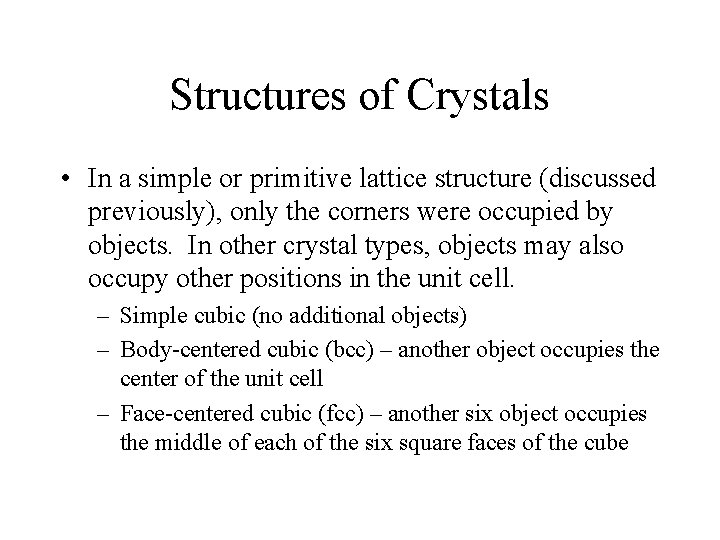Structures of Crystals • In a simple or primitive lattice structure (discussed previously), only the corners were occupied by objects. In other crystal types, objects may also occupy other positions in the unit cell. – Simple cubic (no additional objects) – Body-centered cubic (bcc) – another object occupies the center of the unit cell – Face-centered cubic (fcc) – another six object occupies the middle of each of the six square faces of the cubeStructures of Crystals How many objects/atoms per unit cell for each crystal type? Discuss Look at Figure 13 -24.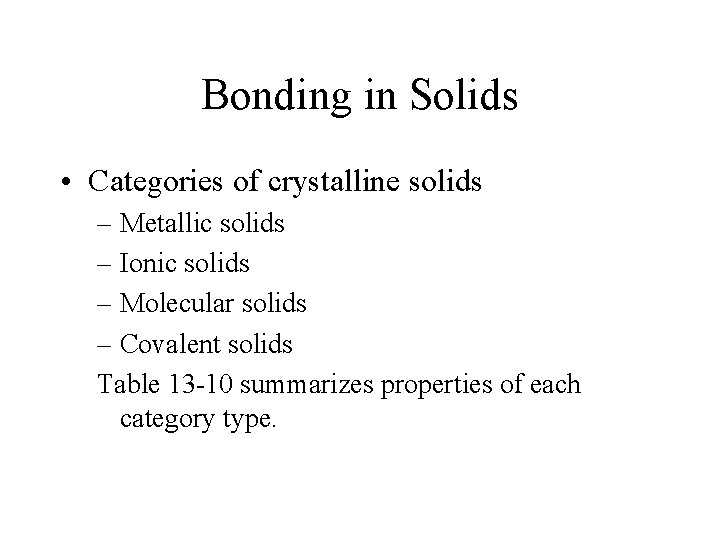Bonding in Solids • Categories of crystalline solids – Metallic solids – Ionic solids – Molecular solids – Covalent solids Table 13 -10 summarizes properties of each category type.Metallic Solids • Positively charged nuclei are surrounded by a sea of delocalized valence electrons. This is the reason why most metals are good conductors. – The nuclei occupy lattice sites • Lattice types for metals – Body-centered cubic (bcc) – Face-centered cubic (fcc) – Hexagonal close-packed (hcp) Examples: Li, K, Ca, and AuMetallic Solids • Obtaining the close-packed structures (fcc and hcp) – Hexagonal close packed structure has an ABA arrangement. • The different letters correspond to different planes (Figure 1327 a). – Face-centered cubic (or cubic close-packed) structure has an ABC arrangement. • Figure 13 -27 b For the close-packed structures approximately 74% of the volume is occupied. The body-centered cubic structures has much less of its volume occupied by metal spheres.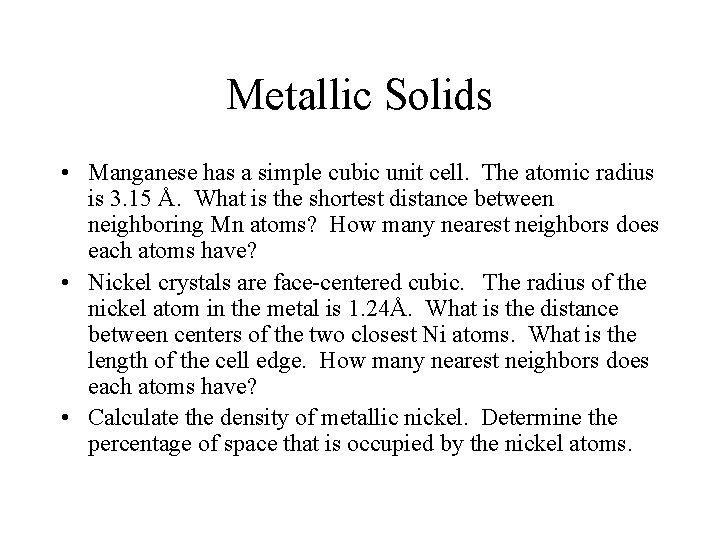Metallic Solids • Manganese has a simple cubic unit cell. The atomic radius is 3. 15 Å. What is the shortest distance between neighboring Mn atoms? How many nearest neighbors does each atoms have? • Nickel crystals are face-centered cubic. The radius of the nickel atom in the metal is 1. 24Å. What is the distance between centers of the two closest Ni atoms. What is the length of the cell edge. How many nearest neighbors does each atoms have? • Calculate the density of metallic nickel. Determine the percentage of space that is occupied by the nickel atoms.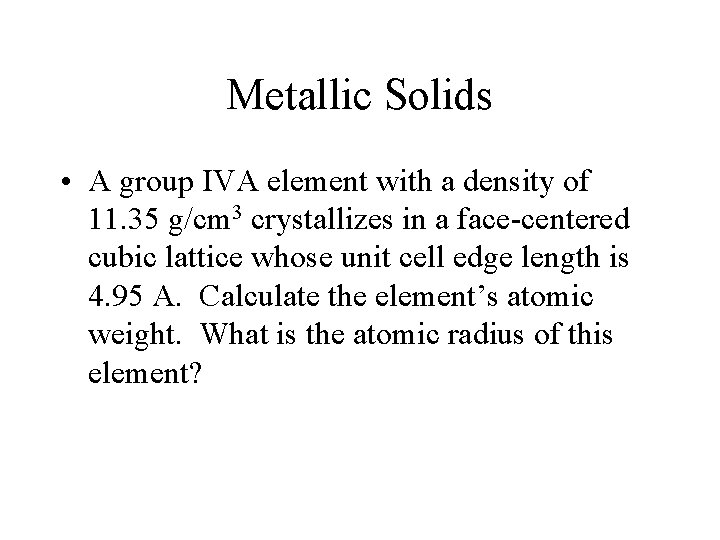Metallic Solids • A group IVA element with a density of 11. 35 g/cm 3 crystallizes in a face-centered cubic lattice whose unit cell edge length is 4. 95 A. Calculate the element’s atomic weight. What is the atomic radius of this element?Ionic Solids • Most salts crystallize as ionic solids with ions occupying the unit cell. The most common example is sodium chloride, which has a face -centered cubic arrangement. – Many other salts that have the same charge on both the anion and cation have the same fcc arrangement (Li. Cl and Mg. O). • Even though the solid compound possesses charge, it does not conduct electricity. Why?Ionic Solids • How many Cl- and Na+ ions are in the fcc unit cell? Remember, a sodium ion is in the center. – Illustrate models and look at 13 -13 on demo CD. Sodium iodide crystallizes in the fcc structure (like Na. Cl). The I- ion radius is 2. 20 Å. The I- ions at the corners of the unit cell are in contact with those at the centers of the faces. Determine the length of the unit cell. Calculate the radius of the Na+ ion assuming anioncation contact.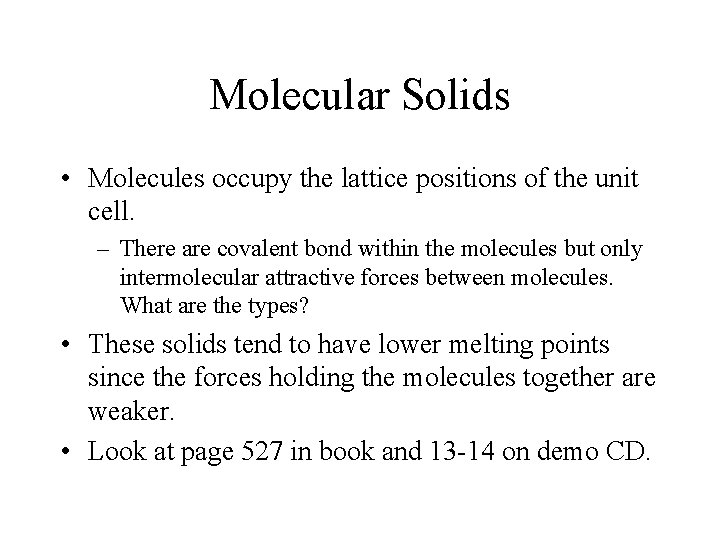Molecular Solids • Molecules occupy the lattice positions of the unit cell. – There are covalent bond within the molecules but only intermolecular attractive forces between molecules. What are the types? • These solids tend to have lower melting points since the forces holding the molecules together are weaker. • Look at page 527 in book and 13 -14 on demo CD.Covalent Solids (‘network solids’) • These can be considered giant molecules with the atom bond covalently in a crystalline network. – Structures are usually very hard with high melting points. Most are poor thermal and electrical conductors. – Examples: Diamond, graphite, and quartz • What is the bonding in diamond (hydridization) and graphite? 13 -15 on demo. CD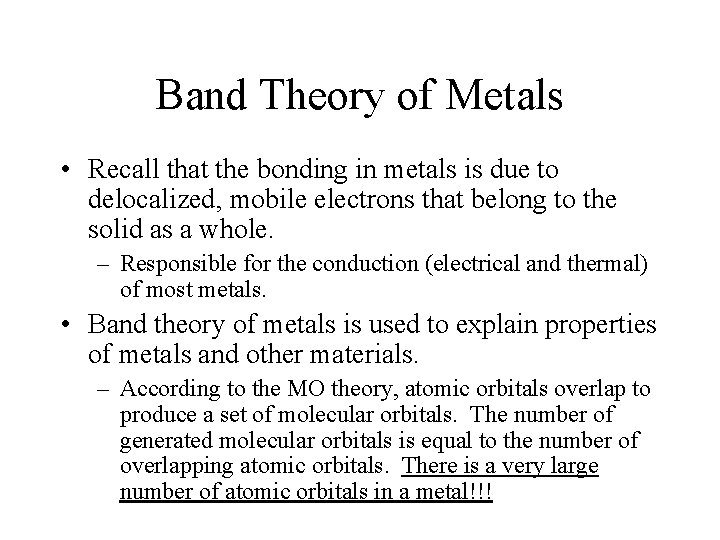Band Theory of Metals • Recall that the bonding in metals is due to delocalized, mobile electrons that belong to the solid as a whole. – Responsible for the conduction (electrical and thermal) of most metals. • Band theory of metals is used to explain properties of metals and other materials. – According to the MO theory, atomic orbitals overlap to produce a set of molecular orbitals. The number of generated molecular orbitals is equal to the number of overlapping atomic orbitals. There is a very large number of atomic orbitals in a metal!!!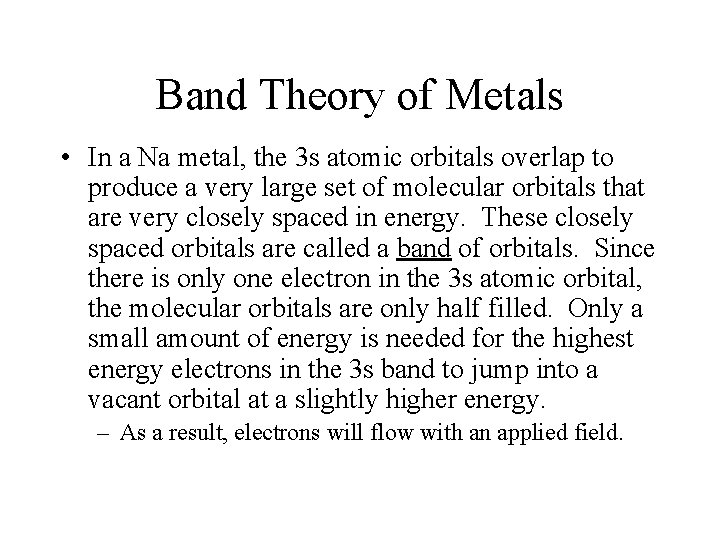Band Theory of Metals • In a Na metal, the 3 s atomic orbitals overlap to produce a very large set of molecular orbitals that are very closely spaced in energy. These closely spaced orbitals are called a band of orbitals. Since there is only one electron in the 3 s atomic orbital, the molecular orbitals are only half filled. Only a small amount of energy is needed for the highest energy electrons in the 3 s band to jump into a vacant orbital at a slightly higher energy. – As a result, electrons will flow with an applied field.Band Theory of Metals What if the 3 s band is filled? In the Group IIA metals, the 3 s band is filled, but the metals still conduct.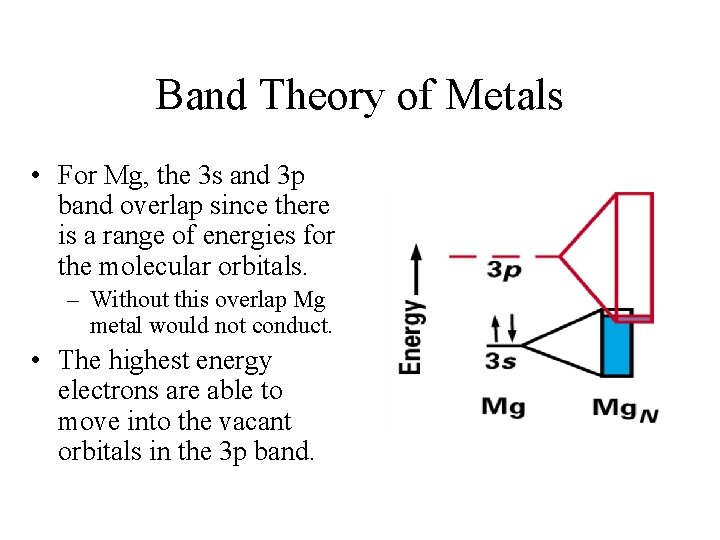Band Theory of Metals • For Mg, the 3 s and 3 p band overlap since there is a range of energies for the molecular orbitals. – Without this overlap Mg metal would not conduct. • The highest energy electrons are able to move into the vacant orbitals in the 3 p band.Band Theory of Metals • Why don’t some materials conduct electricity? – The highest energy electrons in insulators occupy filled bands of molecular orbitals that are well separated from the lowest empty band. – For semiconductors, the filled bands are only slightly below the empty bands. • Generally, increasing the temperature or ‘doping’ the material will produce conduction (page 518 -519).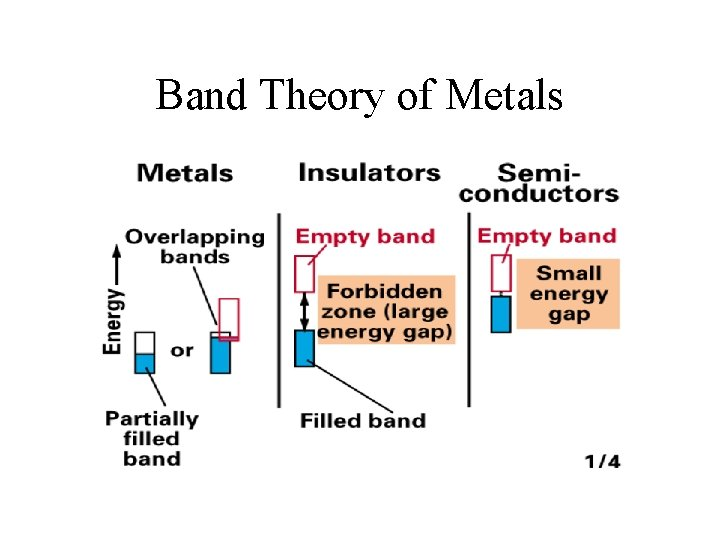Band Theory of Metals